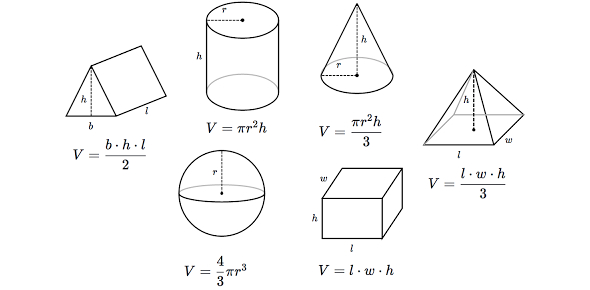# Math Quiz: Geometry MCQ!

10 Questions | Total Attempts: 62Settings.

• 1.
Let F and F' be fields such that |F| = 9, |F'| = 4. the total number of field homomorphism from F to F' is
• A.

2

• B.

1

• C.

0

• D.

3

• 2.
Let V be a vector space of 2*2  matrices over R. Which of the following is a linear transformation.
• A.

T([aij]) = a11

• B.

T(A) = A + I

• C.

T(A) = trace(A)

• D.

I and III only

• 3.
P is orthogonal if and only if PT is orthogonal.
• A.

Yes

• B.

No

• 4.
A cantor set is nowhere dense.
• A.

True

• B.

False

• 5.
Let T : U--> V be a linear transformation , if dim(U) > dim(V) then T is one-one.
• A.

Yes

• B.

No

• 6.
C=2Πr (Circumference of a Circle)
• A.

True

• B.

False

• 7.
Which of the following sets is uncountable?
• A.

Q*Q

• B.

Z*Z

• C.

[0,1] - {1/n : n belong to natural numbers}

• D.

{1/n : n are natural numbers}

• 8.
The total number of subfields of a field with 729 elements is:
• A.

One

• B.

Two

• C.

Three

• D.

Four

• 9.
A finite-dimensional normed space is separable.
• A.

True

• B.

False

• 10.
Which of the following is correct for set  A = {(x,y) : y= 4x } U {(x,y) : x2 + y2 =1}
• A.

A is compact set

• B.

A is connected set

• C.

A is not perfect set

• D.

A is neither open nor closed

Related TopicsBack to top